# Physics Paper 2 Questions and Answers - Form 4 Opener Term 1 Exams 2022

PHYSICS
PAPER TWO

Instructions

• This paper consists of two sections A and B.
• Answer all the questions in the two sections in the spaces provided after each question.
• Electronic calculators and mathematical tables may be used.
• All numerical answers should be expressed in decimal notations.## Questions

SECTION A

1. Two metallic spheres A,B stand in contact as shown. A positively charged rod is held near sphere A. Show the charge on each sphere when the metallic balls are separated and the rod is removed. (2marks)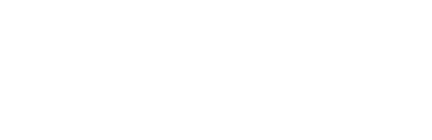2. State two conditions necessary for total internal reflection to occur (2marks)
3.
1. Define the term focal length as applied in the study of curved mirrors (1mark)
2. An object O stands on the principal axis of a concave mirror as shown below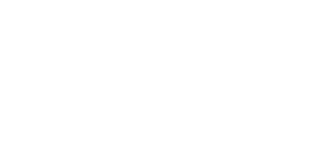1. By drawing suitable rays, show the position of the image (3marks)
2. Determine the magnification of the image formed (2marks)
4. Distinguish between an electromagnetic wave and a mechanical wave (2marks)
5.
1. Explain why repulsion method is the best test for polarity of a magnet as opposed to attraction. (1 mark)
2. A coil of insulated wire is wound around a u – shaped soft iron core X Y and connected to a battery as shown below.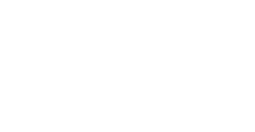State the polarities of the ends X and Y. (2 marks)
6. Loudspeaker placed between two wall A and B is sending out constant wave pulses. Determine how far the loudspeaker is from wall B if it is 100m from wall A, and the time between the two echoes received is o.2 seconds (speed of sound is 340m/s) (3 marks)
7.
1. Explain why eight dry cells in series cannot be used to start a car engine even though they have the same e.m.f. as the lead acid accumulator (1 mark)
8. State two characteristics of images formed by plane mirrors. (2 marks)
9. The figure shows a ray of light incident on the face of a water prism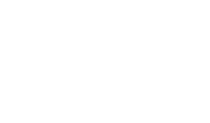Sketch the path of the ray as it passes through the prism .Critical angle for water is 390 (2 marks)
10. A car battery requires topping up with distilled water occasionally. Explain why this is necessary and why distilled water is used. (1 marks)

SECTION B

1.
1. State Ohm’s law (1mark)
2. The diagram below shows a circuit with a coil used to warm oil in a beaker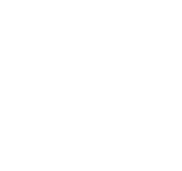1. Explain how heat is produced in the coil (2marks)
2. Given that the reading of the ammeter is 2.4A determine the resistance of the coil. (3marks)
3. How much heat is produced in the coil in a minute? (3mks)
4. Give two changes that can be made in the set up in order to produce more heat per minute (2mks)
2.
1. Define capacitance of a capacitor (1mk)
2. The figure below shows a charged electroscope two aluminum plates A and B arranged a shown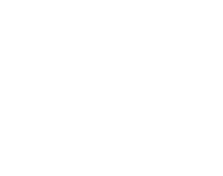State and explain the observations made when:
1. d is reduced (2mk)
2. the plate A is more horizontal (2mks)
3. a sheet of polythene is placed between A and B (2mks)
3. Three capacitors are connected to a 10V battery as shown below.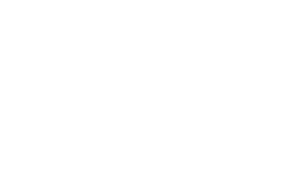1. Calculate the combined capacitance (2 marks)
2. What is the charge on the 3 F capacitor (3marks)
3. p.d across 4f capacitor ( 2 marks)
3.
1. State Snell’s law (1 mark)
2. The figure below shows a ray of light travelling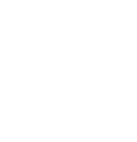1. Calculate the refractive index of water with respect to glass given the refractive index of glass and water are 3/2 and 4/3 respectively. (2 marks)
2. Calculate the angle Ɵ (2 marks)
3. Using a well-labeled diagram, describe how optic fibers are used for communication. (3 marks)
4. A ray of light is incident to a glass block as shown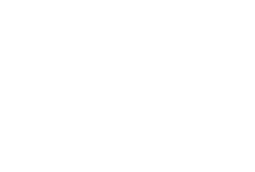Calculate the distance moved by the ray in the block given the refractive index of glass is 1.5. (3mks)
4.
1. In an experiment to study one of the properties of waves, a double slit was placed close to the source of monochromatic light as shown below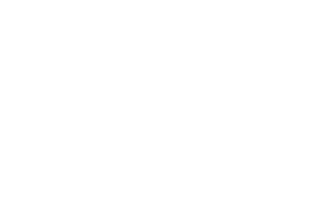1. What property of waves is being investigated? (1 mark)
2. State the function of the double slit. (1 mark)
3. State and explain the observation made on the screen. (2 marks)
4. State what is observed on the screen when;
1. the slit separation S1 S2 is decreased. (1 mark)
2. White source of light is used in place of monochromatic source. (1 mark
3. S1 and S2 are made larger. (1 mark)
2. The diagram below shows plane wave fronts in a ripple tank incident on a boundary between a deep to shallow region.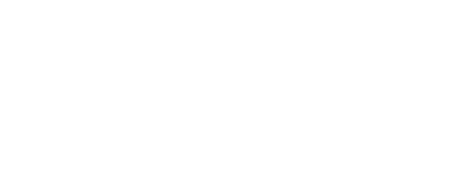On the same diagram, sketch the wave pattern in and beyond the shallow region. (2 marks)
5.
1. Figure 10 shows a graph of 1/v against 1/u for a concave mirror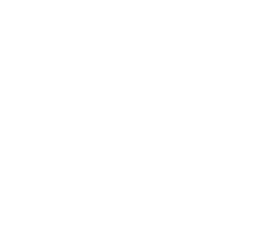1. Use this graph to determine the focal length of the mirror. (2marks)
2. Determine the image distance when the magnification is m = 2 for the concave mirror above. (3 marks)
2. State one application of each of the following
1. Convex mirror. (1mark)
2. Parabolic mirror. (1mark)
3. A small object is placed 15 cm in front of a convex mirror of focal length 10 cm. Determine the position of the image. (3marks)## Marking Scheme

1.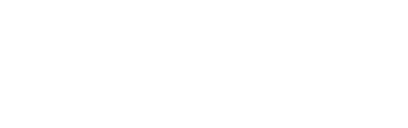2.
• The ray should be moving from a more optically denser median to a less optically denser medium
• The angle of incidence should be greater than the critical angle

3.
1. This is the distance between the focal point and the pole of the mirror

2.
1.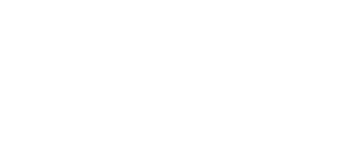2. m= hi/ho = 4.2cm/1.3 cm = 3.231

4. Electromagnetic waves are waves that do not require a medium for propagation while mechanical waves require a medium of propagation
All electromagentic waves are transverse in nature while mechanical waves can be grouped into either transverse or longitudinal

5.
1. Attraction between unlike poles of a magnet and between magnet and magnetic material while repulsion only occures between like poles of magnets.

2. X - Northpole
Y - South pole

6. T1 = 100m x 2
340m/s
T2 =   2x m
340m/s
T2 - T1 = 0.2 sec
2x   200    = 0.2
340       340
2x - 200 = 68
2x  = 268
2       2
x = 134
134m from wall

7.
1. Dry cells have high internal resistance which reduces the amount of current being produced by the cells in series

8.
1. The images are upright
2. Laterally inverted
3. Same size as the object

9.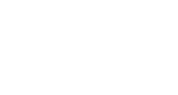10. During charging the water evaporates causing the density of the acid to rise. Distilled water is used to neutralize the acid. Also distilled water is used as it does not have impurities.

11.
1. It states that the current flowing through a conductor is directly proportional to the potential diffrence across it provided the temperature and other physical conditions are kept constant.

2.
1. As the current in flowing through the conditions, it encounters a lot of resistance in the heating coil.
As a result, some of the electrical energy is converted into heat energy (as the electrons collide with the atoms)

2. V= IR
12 = 2.4 x R
R= 12/2.4
=5Ω

3. H = V²t
R
12² x 1 x 60
5
8640
5
= 1728 joules

4.
• Use a high resistance cable i the heating coil
• Increase the amount of current flowing in the circuit
• Increase the length of the heating coil

12.
1.
•  C₁C₂
C₁+C₂
4 x 4
4+4
16/8
2μf
2 + 3
= 5μf

2.
• Q = CV
5 x 10-6 x 10 = 5 x 10-5C
3 x 10-6 x 10
3 x 10-5C

3.
• 1/4 x 1/4 = 2/4 = 2μf
2 x 10-6
5 x 10-5  - 3 x 10-5
2 x 10-5
V= k/c
2  x 10¯⁵
2 x 10-5
= 1

13.
1.
• The ratio of the sine of angle of incidence to the sine of angle of refraction is constant for a given pair of media

2.
1.  n= 3/2
a g
n = 4/3
a w
n  .   n  =  n    x  1/n
a w      a g     a w            a w
n =   n   x  1/n
w g      a w          a w
4/3 x 2/3 = 8/9

2. Sin i = n
Sin r
Sin 30° = 8/9
Sin θ
9/8 x    0.5   = 8/9 x 9/8 Sin t
Sin t
Sin θ = 9/8 x 0.5
Sin-1t = 34.23°
3.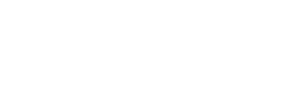A ray of light undegoes repeated total internal reflection on the boundary of the high and low refraction index glass.
4.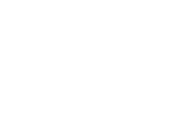Sin i = 1.5
Sin r
Sin 48.6 = 1.5
Sin r
0.7501 = 1.5
Sin r
0.7501 = Sin r
1.5
Sin-1r = 0.5006
r= 30
Cos θ = aff/17y
Hyp = aff/Cos θ
= 10/Cos 3θ
= 11.55cm
14.
1.
1. Interference of light
2. It acts as the coherent source of light
3. It will form dark and bright fringes
Dark fringes is due to destructive interference while bright fringes is due to constructive interference.
4.
1. The separation of fringes is increased.
2. Bright and dark fringes will not form but it will form seven colours of rainbow
3. The separation of fringes is decreased.
2.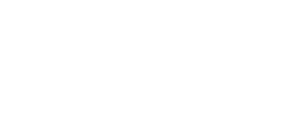15.
1.
1. 1/v = 1/5 = 1/0.2 = 10/2 = 2
1/u = 1/f = 1/0.2 = 10/2
1/5 = 10/2
5= 2/10
= 0.2 + 0.2
2
= 0.1

2. V/5 = 1+ m
V/5 = 1 + 2
v/0.2 = 112
V= 3 x 0.1
= 0.3cm

• ✔ To read offline at any time.
• ✔ To Print at your convenience
• ✔ Share Easily with Friends / Students